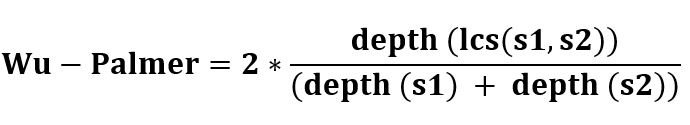# NLP | WuPalmer – WordNet Similarity

• Last Updated : 25 Jul, 2022

How does Wu & Palmer Similarity work
It calculates relatedness by considering the depths of the two synsets in the WordNet taxonomies, along with the depth of the LCS (Least Common Subsumer).The score can be 0 < score <= 1. The score can never be zero because the depth of the LCS is never zero (the depth of the root of taxonomy is one).
It calculates the similarity based on how similar the word senses are and where the Synsets occur relative to each other in the hypernym tree.
Code #1: Introducing Synsets

## Python3

 `from` `nltk.corpus ``import` `wordnet` `syn1 ``=` `wordnet.synsets(``'hello'``)[``0``]``syn2 ``=` `wordnet.synsets(``'selling'``)[``0``]` `print` `(``"hello name :  "``, syn1.name())``print` `(``"selling name :  "``, syn2.name())`

Output :

```hello name :   hello.n.01
selling name :   selling.n.01```

Code #2: Wu Similarity

## Python3

 `syn1.wup_similarity(syn2)`

Output :

`0.26666666666666666`

hello and selling is apparently 27% similar! This is because they share common hypernyms further up the two.

Code #3: Let’s check the hypernyms in between.

## Python3

 `sorted``(syn1.common_hypernyms(syn2))`

Output :

`[Synset('abstraction.n.06'), Synset('entity.n.01')]`

One of the core metrics used to calculate similarity is the shortest path the distance between the two Synsets and their common hypernym.

Code #4: Let’s understand the use of hypernym.

## Python3

 `ref ``=` `syn1.hypernyms()[``0``]``print` `(``"Self comparison : "``,``       ``syn1.shortest_path_distance(ref))` `print` `(``"Distance of hello from greeting : "``,``       ``syn1.shortest_path_distance(syn2))` `print` `(``"Distance of greeting from hello : "``,``       ``syn2.shortest_path_distance(syn1))`

Output :

```Self comparison :  1
Distance of hello from greeting :  11
Distance of greeting from hello :  11```

Note: The similarity score is very high i.e. they are many steps away from each other because they are not so similar. The codes mentioned here use ‘noun’ but one can use any Part of Speech (POS).

My Personal Notes arrow_drop_up